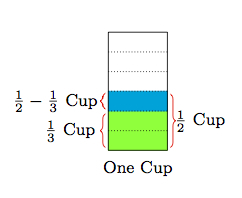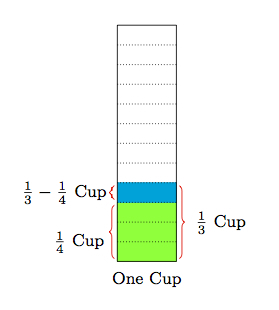# Measuring Cups

Alignments to Content Standards: 5.NF.A

Lucy has measuring cups of sizes 1 cup, $\frac{1}{2}$ cup, $\frac{1}{3}$ cup, and $\frac{1}{4}$ cup. She is trying to measure out $\frac{1}{6}$ of a cup of water and says, ''If I fill up the the $\frac{1}{2}$ cup and then pour that into the $\frac{1}{3}$ cup until it is full, there will be $\frac{1}{6}$ of a cup of water left.''

1. Is Lucy's method to measure $\frac{1}{6}$ of a cup of water correct? Explain.
2. Lucy wonders what other amounts she can measure. Is it possible for her to measure out $\frac{1}{12}$ of a cup? Explain.
3. What other amounts of water can Lucy measure?

## IM Commentary

This task could form part of a classroom activity where students are encouraged to find as many different ways as possible to make different fractions such as $\frac{1}{12}$ and then share their methods. While measuring cups would be helpful to experiment with and explore the different possibilities of how to use the cups, students will need to use mathematics in order to calculate the precise amount of water left in any given cup.

Part (c) of the question has been deliberately left vague. On the one hand, students might provide one other amount that Lucy can measure, such as 7/12 of a cup which they can make by combining 1/3 of a cup and 1/4 of a cup in the one cup container. On the other hand, they could attempt to find all possible amounts that Lucy can measure, experimenting with different possible combinations, making lists, and sharing their ideas. Finally, for advanced students, they might be challenged to produce a complete list and explain why it is complete (some indications how to do this are provided in the solution).

The mathematics here is very closely related to work with Egyptian fractions (see for example www.illustrativemathematics.org/illustrations/839). Egyptian fractions, however, are sums of unit fractions while this work with measuring cups gives both sums and differences. Moreover, Lucy can use the same measuring cup multiple times. A different variant on this task, which would capture the spirit of Egyptian fractions, would allow Lucy to use each measuring cup at most a single time.

Work on this task can support many of the standards of mathematical practice including

• MP2, Reason Abstractly and Quantitatively: students need to find a common denominator to calculate what happens as water is poured from one cup to another and the third part of the question requires analyzing the situation from an abstract viewpoint.
• MP3, Construct viable arguments and critique the reasoning of others: part (c) of the question encourages students to create other fractions using the measuring cup model. They will need to explain why their method works and, if done as a group activity, can share and critically discuss their numbers and methods. The more deeply students go into part (c), the richer the opportunities will be to engage in MP3.
• MP4, Model With Mathematics: students must describe with fractions what happens when water is poured from one measuring cup into another.

## Solution

1. Lucy starts out with $\frac{1}{2}$ cup of water in one container and then uses that to fill up the $\frac{1}{3}$ cup container. This means that there will be $\frac{1}{2} - \frac{1}{3}$ cups left in the $\frac{1}{2}$ cup container. We can use 6 as a common denominator to calculate this fraction:

\begin{align} \frac{1}{2} - \frac{1}{3} &= \frac{1}{2} \times \frac{3}{3} - \frac{1}{3} \times \frac{2}{2} \\ &= \frac{3}{6} - \frac{2}{6} \\ &= \frac{1}{6}. \end{align}

So there is $\frac{1}{6}$ of a cup of water left in the $\frac{1}{2}$ cup container.

Below is a way to picture thisThe different measuring cups are not pictured but we can see $\frac{1}{2}$ of a full cup shaded in both green and blue while $\frac{1}{3}$ of a cup is shaded in green. So after pouring $\frac{1}{3}$ of a cup out of the $\frac{1}{2}$ cup container what will remain is in blue. The full cup is divided into six equal pieces and one of them is blue. This means that $$\frac{1}{2} - \frac{1}{3} = \frac{1}{6}$$

and $\frac{1}{6}$ of a cup of water remains in the $\frac{1}{2}$ cup container.

2. One way Lucy can measure out $\frac{1}{12}$ of a cup is to follow the same method as in part (a) except this time pour water from the $\frac{1}{3}$ cup container into the $\frac{1}{4}$ cup container. Once the smaller container is full, there will be $\frac{1}{3} - \frac{1}{4}$ cups of water left in the $\frac{1}{3}$ cup container. We calculate this amount as we did in part (a):

\begin{align} \frac{1}{3} - \frac{1}{4} &= \frac{1}{3} \times \frac{4}{4} - \frac{1}{4} \times \frac{3}{3} \\ &= \frac{4}{12} - \frac{3}{12} \\ &= \frac{1}{12}. \end{align}

So there will be $\frac{1}{12}$ of a cup of water left in the $\frac{1}{3}$ cup container. This is pictured in two steps below:

With pictures, in order to show both $\frac{1}{3}$ and $\frac{1}{4}$ of a cup we draw one cup, divided into 12 equal pieces:This shows that after pouring $\frac{1}{4}$ of a cup out of the $\frac{1}{3}$ cup container, $\frac{1}{12}$ of a cup will remain.

3. We have seen in parts (a) and (b) that Lucy can measure $\frac{1}{6} = \frac{2}{12}$ of a cup and also $\frac{1}{12}$ of a cup. Her three containers measure $\frac{1}{4} = \frac{3}{12}$ of a cup, $\frac{1}{3} = \frac{4}{12}$ of a cup, and $\frac{1}{2} = \frac{6}{12}$ of a cup. For $\frac{5}{12}$ of a cup, she can measure $\frac{1}{12}$ of a cup and put this in her $\frac{1}{2}$ cup container: then she can fill up the $\frac{1}{3} = \frac{4}{12}$ cup container and add this giving $\frac{5}{12}$ of a cup. In order to get $\frac{7}{12}$, $\frac{8}{12}$, $\frac{9}{12}$, $\frac{10}{12}$, $\frac{11}{12}$, and $\frac{12}{12}$ of a cup, Lucy can add $\frac{1}{2} = \frac{6}{12}$ to the one cup container and then measure out the appropriate number of twelfths of a cup (1,2,3,4, 5, or 6) using the other containers and then add this to the one cup container.

Lucy can only measure a whole number of twelfths of a cup--for example, she could measure $\frac{17}{12} = 1\frac{5}{12}$--with the cups she has. To see why, notice that each cup measures a whole number of $\frac{1}{12}$ cups. Pouring water out of one container and into another allows her to add or subtract these amounts. Adding and subtracting a whole number of twelfths will always lead to a whole number of twelfths.